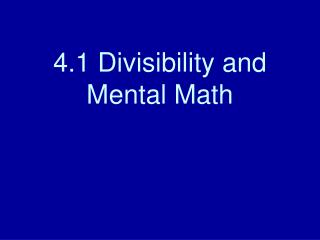Download Presentation4.1 Divisibility and Mental Math

# 4.1 Divisibility and Mental Math - PowerPoint PPT PresentationDownload Presentation## 4.1 Divisibility and Mental Math

- - - - - - - - - - - - - - - - - - - - - - - - - - - E N D - - - - - - - - - - - - - - - - - - - - - - - - - - -
##### Presentation Transcript

1. 4.1 Divisibility and Mental Math

2. Mental Math • Is 56 divisible by 7? Think! • 56 = 8 x 7 • Is 56 divisible by 4? Think! • 56 = 8 x 7, and 4 x 2 = 8, 56 is divisible by 4.

3. Mental Math • Is 64 divisible by 6? • No • Is 93 divisible by 3? • Yes

4. Divisibility Rules • A whole number is divisible by • 2, if the number ends in 0, 2, 4, 6, or 8. • 3, if the sum of the number’s digits is divisible by 3. • 5, if the number ends in 0 or 5 • 9, if the sum of the number’s digits is divisible by 9 • 10, if the number ends in 0.

5. Divisibility by 2, 5, 10 • Ends in … • Test each number for divisibility by 2, 5, 10 • 150 • 1,021 • 2,112 • 65 • 100

6. Divisibility by 3 • Sum of number’s digits is divisible by 3 • 715 • 7 + 1 + 5 = 13 • 13 is not divisible by 3 • 150 • 1 + 5 + 0 = 6 • 6 is divisible by 3

7. Divisibility by 9 • sum of number’s digits is divisible by 9 • 163 • 1 + 6 + 3 = 10 • 10 is not divisible by 9 • 126 • 1 + 2 + 6 = 9 • 9 is divisible by 9

8. Try it! • Test each number for divisibility of 2, 3, 5, 9, and 10 • 60 • 48 • 81